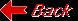Planet UranusDiscovered by William Herschel Date of discovery 1781 Mass (kg) 8.686e+25 Mass (Earth = 1) 1.4535e+01 Equatorial radius (km) 25,559 Equatorial radius (Earth = 1) 4.0074 Mean density (gm/cm^3) 1.29 Mean distance from the Sun (km) 2,870,990,000 Mean distance from the Sun (Earth = 1) 19.1914 Rotational period (hours) -17.9 Orbital period (years) 84.01 Mean orbital velocity (km/sec) 6.81 Orbital eccentricity 0.0461 Tilt of axis (degrees) 97.86 Orbital inclination (degrees) 0.774 Equatorial surface gravity (m/sec^2) 7.77 Equatorial escape velocity (km/sec) 21.30 Visual geometric albedo 0.51 Magnitude (Vo) 5.52 Mean cloud temperature -193C Atmospheric pressure (bars) 1.2 Atmospheric composition: Hydrogen 83%, Helium 15%, Methane 2%